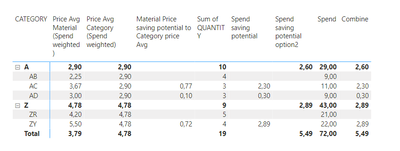cancel
Showing results for
Did you mean:Frequent Visitor

## Computing totals by summing visible values (based on measure) row by row in DAX

Hi,

I have a issue to calculate a measure in a way that it gives the result on row level and on total level in the same measure.

I have managed to calculate two different measures, neither of those give the proper putcome.

Spend saving potential =
CALCULATE(
SUMX('Table', 'Table'[QUANTITY])
[Material Price saving potential to Category price Avg]  )

Spend saving potential option2 =
SUMX(
VALUES('Table'[Material] ),
IF([Spend saving potential] >0, [Spend saving potential])
)

As can be seen in the picture below, the "Spend saving potential" is the aggregated savings of materials where the material average price is over the correspondedcategory averages. If material prices are under category avg prices, those are blanked out.Thanks if you can solve this!

1 ACCEPTED SOLUTIONSolution Sage

Thank you for direct sharing your PBI. I make it more easier for helping.
Try this

`Combine = VAR _cat = CALCULATE(SUMX('Table', 'Table'[QUANTITY])*[Material Price saving potential to Category price Avg])VAR _mat = SUMX(    VALUES('Table'[Material]),    IF([Spend saving potential] >0, [Spend saving potential]))RETURNIF(HASONEFILTER('Table'[Material]),_cat,_mat)`4 REPLIES 4Frequent Visitor

Hi,

The proposed solution was good enough for the time being, so I accepted yi as solution.

Thank you @andhiii079845 !Solution Sage

I have to look later in the pbi again to answer your question in general you want to combine to different measures which have different filters and one a "group by" per 'Table'[Material] . So you have to decide what you want to show when.

`In other words, I am lacking understanding why "Spend saving potential" does not produce value for total? And also lacking understanding why "option2" does not give values for matrix rows.`

Do you mean no totals of the level of the Category? Can you explain more in detail what do you not understand, please? I think (not 100 % sure) you have blank values in the measure ([Material Price saving potential to Category price Avg]) so he can not give a total back for the category or total.Solution Sage

Thank you for direct sharing your PBI. I make it more easier for helping.
Try this

`Combine = VAR _cat = CALCULATE(SUMX('Table', 'Table'[QUANTITY])*[Material Price saving potential to Category price Avg])VAR _mat = SUMX(    VALUES('Table'[Material]),    IF([Spend saving potential] >0, [Spend saving potential]))RETURNIF(HASONEFILTER('Table'[Material]),_cat,_mat)`Frequent Visitor

Hi,

In a way, this resolve the case.

However I wonder is there more "sophicticated / simplified" measure, which would automatically calculate desired outcome (one code for rows and also for totals) without using this kind of "workaround" where the code is longer and more complicated.
In other words, I am lacking understanding why "Spend saving potential" does not produce value for total? And also lacking understanding why "option2" does not give values for matrix rows.

Thanks for support!